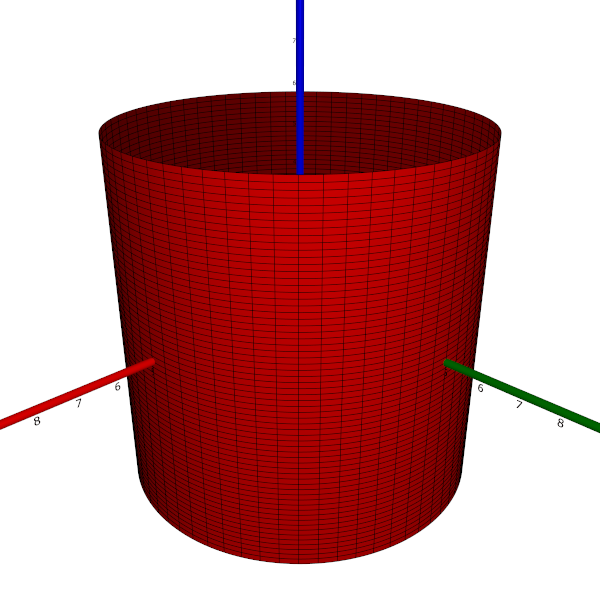$\newcommand{\sech}{\mathop{\rm sech}\nolimits} \newcommand{\csch}{\mathop{\rm csch}\nolimits} \newcommand{\R}{\mathbb{R}} \newcommand{\basis}{\underline{\mathbf{e}}}$
Current Page
Elliptic cylinder

# Elliptic cylinder

Object type: Surface

### Definition

In $\mathbb{R}^3$, an elliptic cylinder is a set of points $(x, y, z)$ satisfying the equation $$\left(\frac{x}{a}\right)^2 + \left(\frac{y}{b}\right)^2 = 1$$ for some constants $a, b > 0$. If $a = b ≕ r$, the surface is a circular cylinder of radius $r$. Below, a part of a circular cylinder of radius $r = 5$ is shown.Each intersection with a plane $z = c$ is an ellipse, and all such intersections are identical except for a translation in the $z$ direction. This defines the elliptic cylinder.

### Parameterisation

The elliptic cylinder is the image $\mathbf{r}([0, 2π[×\mathbb{R})$ where $$\mathbf{r}(u, v) = \underline{\mathbf{e}}\begin{pmatrix}a\cos{u}\\b\sin{u}\\v\end {pmatrix}.$$

### Properties

The following properties are with respect to the parameterisation given above.

#### Parameter-curve tangent vectors

The parameter-curve tangent vectors are $$\mathbf{r}_u(u,v)=\underline{\mathbf{e}} \begin{pmatrix}-a\sin{u}\\b\cos{u}\\0\end{pmatrix}, \quad\quad \mathbf{r}_v(u,v)= \underline{\mathbf{e}}\begin{pmatrix}0\\0\\1\end{pmatrix}.$$

#### Standard unit normal

The standard unit normal vector field is $$\mathbf{\hat{N}}(u,v) = \frac{1}{\sqrt{a^2 \sin^2{u} + b^2\cos^2{u}}}\underline{\mathbf{e}}\begin{pmatrix}b\cos{u}\\a\sin{u}\\0 \end{pmatrix}.$$ In the special case of a circular cylinder, this reduces to $$\mathbf{\hat{N}} (u,v) = \underline{\mathbf{e}}\begin{pmatrix}\cos{u}\\\sin{u}\\0\end{pmatrix} .$$

#### Area element

The area element is $$dA = \sqrt{a^2\sin^2{u} + b^2\cos^2{u}}~dudv.$$ In the particular case of a circular cylinder, this reduces to $dA = r~dudv$.

#### First fundamental form

The first fundamental form of the elliptic cylinder is $$\mathcal{F}(u,v)=\begin{pmatrix} a^2\sin^2{u} + b^2\cos^2{u}&&0\\0&&1\end{pmatrix}.$$ In the special case of a circular cylinder, this reduces to $$\mathcal{F}(u,v) = \begin{pmatrix}r^2&&0\\0&&1\end{pmatrix}.$$

#### Second fundamental form

The second fundamental form of the elliptic cylinder is $$\mathcal{M}(u,v) = \begin {pmatrix}-\frac{ab}{\sqrt{a^2\sin^2{u} + b^2\cos^2{u}}}&&0\\0&&0\end{pmatrix},$$ which, in the case of a circular cylinder, reduces to $$\mathcal{M}(u,v) = \begin{pmatrix}-r&&0\\0&& 0\end{pmatrix}.$$

#### Christoffel symbols

The Christoffel symbols for the elliptic cylinder are $$\Gamma^1_{\alpha\beta} = \begin {pmatrix}\frac{(a^2-b^2)\sin{2u}}{2a^2\sin^2 u + 2b^2\cos^2 u}&&0\\0&&0\end{pmatrix}, \quad\quad \Gamma^2_{\alpha\beta} = \begin{pmatrix}0&&0\\0&&0\end{pmatrix}.$$ In particular, for the circular cylinder, these reduce to $$\Gamma^1_{\alpha\beta} = \begin{pmatrix}0&&0\\0&&0\end{pmatrix}, \quad \quad\Gamma^2_{\alpha\beta} = \begin{pmatrix}0&&0\\0&&0\end{pmatrix}.$$

#### Curvatures

The principal curvatures of the elliptic cylinder are $$\lambda_1 = -\frac{ab}{\left(a^2 \sin^2{u} + b^2\cos^2{u}\right)^{3/2}}, \quad\quad \lambda_2 = 0$$ and the corresponding principal directions are $(1, 0)$ and $(0, 1)$, respectively. Hence, the Gaussian and mean curvatures are $$K = 0, \quad\quad H = -\frac{ab}{\left(a^2\sin^2{u} + b^2\cos^2{u}\right)^{3/2}}.$$ In the special case of a circular cylinder, the above formulae reduce to $$\lambda_1 = -\frac{1}{r}, \quad\quad \lambda_2 = 0$$ and $$K = 0, \quad\quad H = -\frac{1}{r}.$$